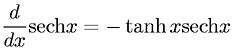Equations > Calculus > Differentiation/Differentals > Derivative of Hyperbolic Secant

### Derivative of Hyperbolic SecantLatex Code:

MathML Code:

 $\fracd\mathrm{dx}\mathrm{sech}\nolimitsx=-\mathrm{tanh}x\mathrm{sech}\nolimitsx$

MathType 5.0: